## Tuesday, December 27, 2016

### Bernoulli vs Binomial vs Multinoulli vs Multinomial distributions

Bernoulli distribution
Say you are flipping a coin that has a probability of 0.4 of turning up heads and 0.6 of turning up tails. The simplest probability distribution there is is the Bernoulli distribution which just asks for the probability of the coin turning up heads after one toss, that is, 0.4. That's all. The Bernoulli distribution is only about the probability of a random event with two possible outcomes occurring after one trial. It's used for success/fail type random variables where you want to reason about the probability that a random variable will succeed (such as a coin turning up heads).

The probability mass function for the Bernoulli distribution is:
f(x;p) = p^x * (1 - p)^(1-x)

where "*" is multiplication and "^" is power, "p" is the probability of a success and "x" is the number of successes desired, which can be either 1 or 0. When "x" is 1 then you find the probability of a success whilst when it is 0 then you find the probability of a fail. Since there are only two outcomes, if "p" is the probability of a success then the probability of a non-success is "1-p". Notice that this function is quite silly really as it is just a closed form expression for choosing either "p" or "1-p" depending on what "x" is. The number you're interested in is raised to the power of 1 whilst the number you're not interested in is raised to the power of 0, turning it into 1, and then the two results are multiplied together.

Binomial distribution

The probability mass function for the Binomial distribution is:
f(x;n,p) = n!/(x! * (n-x)!) * p^x * (1 - p)^(n-x)

where "*" is multiplication and "^" is power, "p" is the probability of a success, "n" is the number of trials to try out, and "x" is the number of desired successes out of the "n" trials.

Multinoulli distribution
Whereas the binomial distribution generalises the Bernoulli distribution across the number of trials, the multinoulli distribution generalises it across the number of outcomes, that is, rolling a dice instead of tossing a coin. The multinoulli distribution is also called the categorical distribution.

The probability mass function for the multinoulli distribution is:
f(xs;ps) = ps_1^xs_1 * ps_2^xs_2 * ... * ps_k^xs_k

where "*" is multiplication and "^" is power, "k" is the number of outcomes (6 in the case of a dice, 2 for a coin), "ps" is the list of "k" probabilities where "ps_i" is the probability of the ith outcome resulting in a success, "xs" is a list of numbers of successes where "xs_i" is the number of successes desired for the ith outcome, which can be either 1 or 0 and where there can only be exactly a single "1" in "xs".

Multinomial distribution
Finally the most general generalisation of the Bernoulli distribution is across both the number of trials and the number of outcomes, called the multinomial distribution. In order to be more general, this distribution also allows specifying the number of successes desired for each individual outcome, rather than just of one outcome like the multinoulli distribution. This lets us calculate the probability of rolling a dice 6 times and getting 3 "2"s, 2 "3"s and a "5". Since we want it to be compatible with the binomial distribution, these outcomes can arrival in any order, that is, it doesn't matter whether you get [5,3,2,3,2,2] or [2,2,2,3,3,5]. For this reason we need to use the combination function again.

The probability mass function for the multinomial distribution is:
f(xs;n,ps) = n!/(xs_1! * xs_2! * ... * xs_k!) * ps_1^xs_1 * ps_2^xs_2 * ... * ps_k^xs_k

where "*" is multiplication and "^" is power, "k" is the number of outcomes (6 in the case of a dice, 2 for a coin), "ps" is the list of "k" probabilities where "ps_i" is the probability of the ith outcome resulting in a success, "xs" is a list of numbers of successes where "xs_i" is the number of successes desired for the ith outcome, the total of which must be "n".

## Wednesday, November 30, 2016

### Checking if a number is prime: when to stop checking potential factors

Let's say you want to find out whether the number 888659800715261 is a prime number. If this were a programming exercise, a naive solution would be to go through all the numbers from 2 to 888659800715261/2 (half the number) and check whether any of the numbers divide 888659800715261 evenly. If none of them do, then the number is prime. The reason why we stop at half the number is because the largest factor that a number "n" can have before "n" itself is n/2, the one before that is n/3, and so on. Sure, we don't need to check every single number between 2 and n/2 as it would be enough to only check the prime numbers between 2 and n/2, but the point is that as soon as we reach n/2, we can stop checking and declare that 888659800715261 is prime.

The problem is that half of 888659800715261 is 444329900357630 which is still too big to check each number between 2 and it, probably even if you only check the prime numbers. The question is, is there an even lower upper-bound where we can stop checking potential factors in order to be sure that the number has no factors?

Let's look at which numbers between 1 and 24 are factors of 24:
    5  7  9 10 11  13 14 15 16 17 18 19 20 21 22 23 

In order for a number to be a factor of 24, there must be another factor which when the two factors are multiplied together give 24. For example, if 2 is a factor of 24, then there must be another factor of 24 which when multiplied by 2 gives 24. This other factor is 12. Let's call 2 and 12 co-factors. The co-factor of 3 is 8, the co-factor of 4 is 6. We can imagine co-factors being mirror reflections of each other:

    5  7  9 10 11  13 14 15 16 17 18 19 20 21 22 23 
(   [   {   <     >     }           ]                                     )


Notice how all the co-factors nest each other. The co-factors 4 and 6 are between the co-factors 3 and 8 which in turn are between the co-factors 2 and 12 which in turn are between 1 and 24. It seems like there is a line of reflection at 5, where all the factors smaller than 5 have co-factors larger than 5. This means that beyond 5, all factors are co-factors of smaller numbers.

Let's see another list of factors for 16:
  3  5 6 7  9 10 11 12 13 14 15 
(   [    { }        ]                       )


Notice how 4 is a co-factor with itself in this case, since 4 squared is 16. The line of reflection is crossed at 4 this time, which happens to be the square root of 16. In fact the square root of 24 is 4.898... which is close to 5 (the line of reflection of 24). This is always the case, because the line of reflection occurs where the two co-factors are the same. If the co-factors are not the same then one factor must be smaller than the square root whilst the other must be larger. If both are smaller or bigger then when multiplied together they will not give the number being factored. This is because if two co-factors "a" and "b" are supposed to equal "n", and both of them are larger than √n, then that would mean that a×b must also be larger than √n×√n, which means that a×b is larger than "n". The area of a rectangle with two large sides cannot be equal to the area of a rectangle with two small sides. Therefore, if the two sides are equal (a square) then in order to change the length of the sides without changing the area of the rectangle you must make one side larger whilst making the other smaller. This proves that the square root must be between any two co-factors.

So what's the point? The point is that if you reach the square root of "n" without having found any factors, then you are guaranteed that there will not be any factors beyond √n because if there are any then they must have co-factors smaller than √n, which you have already confirmed that there aren't any. So the square root of a number is the lower upper-bound to check for factors. Can there be an even lower upper-bound? No, because the number might be a square number and have no other factors apart from its square root, such as 25. Therefore the smallest co-factor a number can have is the square root of the number.

So in order to check if 888659800715261 is a prime number, we only have to check all the numbers up to 29810397 for factors, which is much better than 444329900357630. By the way, there is no number between 2 and 29810397 that evenly divides 888659800715261, which means that it is a prime number.

## Sunday, October 30, 2016

### Using beam search to generate the most probable sentence

This blog post continues in a second blog post about how to generate the top n most probable sentences.

In my last blog post I talked about how to generate random text using a language model that gives the probability of a particular word following a prefix of a sentence. For example, given the prefix "The dog", a language model might tell you that "barked" has a 5% chance of being the next word whilst "meowed" has a 0.3%. It's one thing generating random text in a way that is guided by the probabilities of the words but it is an entirely different thing to generate the most probable text according to the probabilities. By most probable text we mean that if you multiply the probabilities of all the words in the sentence together you get the maximum product. This is useful for conditioned language models which give you different probabilities depending on some extra input, such as an image description generator which accepts an image apart from the prefix and returns probabilities for the next word depending on what's in the image. In this case we'd like to find the most probable description for a particular image.

You might think that the solution is to always pick the most probable word and add it to the prefix. This is called a greedy search and is known to not give the optimal solution. The reason is because it might be the case that every combination of words following the best first word might not be as good as those following the second best word. We need to use a more exploratory search than greedy search. We can do this by thinking of the problem as searching a probability tree like this: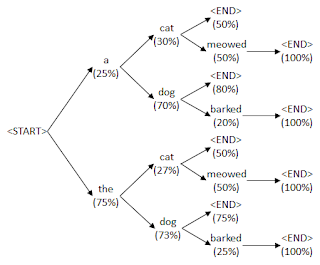The tree shows a probability tree of which words can follow a sequence of words together with their probabilities. To find the probability of a sentence you multiply every probability in the sentence's path from <start> to <end>. For example, the sentence "the dog barked" has a probability of 75% × 73% × 25% × 100% = 13.7%. The problem we want to solve is how to find the sentence that has the highest probability.

One way to do this is to use a breadth first search. Starting from the <start> node, go through every node connected to it, then to every node connected to those nodes and so on. Each node represents a prefix of a sentence. For each prefix compute its probability, which is the product of all the probabilities on its path from the <start> node. As soon as the most probable prefix found is a complete sentence, that would be the most probable sentence. The reason why no other less probable prefixes could ever result in more probable sentences is because as a prefix grows, its probability shrinks. This is because any additional multiplications with probabilities made to any prefix probability will only make it smaller. For example, if a prefix has a probability of 20% and another word is added to it which has a probability of 99%, then the new probability will be 20% × 99% which is the smaller probability of 19.8%.

Of course a breadth first search is impractical on any language model that has a realistic vocabulary and sentence length since it would take too long to check all the prefixes in the tree. We can instead opt to take a more approximate approach where we only check a subset of the prefixes. The subset would be the top 10 most probable prefixes found at that point. We do a breadth first search as explained before but this time only the top 10 most probable prefixes are kept and we stop when the most probable prefix in these top 10 prefixes is a complete sentence.

This is practical but it's important that the way we find the top 10 prefixes is fast. We can't sort all the prefixes and choose the first 10 as there would be too many. We can instead use a heap data structure. This data structure is designed to quickly take in a bunch of numbers and quickly pop out the smallest number. With this you can insert the prefix probabilities one by one until there are 10 prefixes in it. After that start popping out the smallest probability immediately after inserting a new one in order to only keep the 10 largest ones.

Python provides a library called "heapq" (heap queue) just for this situation. Here is a class that makes use of heapq in order to create a beam of prefix probabilities:

import heapq

class Beam(object):
#For comparison of prefixes, the tuple (prefix_probability, complete_sentence) is used.
#This is so that if two prefixes have equal probabilities then a complete sentence is preferred over an incomplete one since (0.5, False) < (0.5, True)

def __init__(self, beam_width):
self.heap = list()
self.beam_width = beam_width

heapq.heappush(self.heap, (prob, complete, prefix))
if len(self.heap) > self.beam_width:
heapq.heappop(self.heap)

def __iter__(self):
return iter(self.heap)


The code to perform the actual beam search is this:

def beamsearch(probabilities_function, beam_width=10, clip_len=-1):
prev_beam = Beam(beam_width)
while True:
curr_beam = Beam(beam_width)

#Add complete sentences that do not yet have the best probability to the current beam, the rest prepare to add more words to them.
for (prefix_prob, complete, prefix) in prev_beam:
if complete == True:
else:
#Get probability of each possible next word for the incomplete prefix.
for (next_prob, next_word) in probabilities_function(prefix):
if next_word == '<end>': #if next word is the end token then mark prefix as complete and leave out the end token
else: #if next word is a non-end token then mark prefix as incomplete

(best_prob, best_complete, best_prefix) = max(curr_beam)
if best_complete == True or len(best_prefix)-1 == clip_len: #if most probable prefix is a complete sentence or has a length that exceeds the clip length (ignoring the start token) then return it
return (best_prefix[1:], best_prob) #return best sentence without the start token and together with its probability

prev_beam = curr_beam


"probabilities_function" returns a list of word/probability pairs given a prefix. "beam_width" is the number of prefixes to keep (so that instead of keeping the top 10 prefixes you can keep the top 100 for example). By making the beam search bigger you can get closer to the actual most probable sentence but it would also take longer to process. "clip_len" is a maximum length to tolerate, beyond which the most probable prefix is returned as an incomplete sentence. Without a maximum length, a faulty probabilities function which does not return a highly probable end token will lead to an infinite loop or excessively long garbage sentences.

## Wednesday, September 28, 2016

### Text generation using a language model

A language model is something that tells you the probability of a sequence of words. For example it tells you that the sequence "a dog is barking" is more probable than "a dog is was". This probability can then be used to generate text by selecting a sequence of words that is probable.

One way to do this is to take a huge amount of text and count how often each 4 word segment occurs, then divide by the total amount of 4 word segments. For example, you find that in your huge text, "a dog is barking" to occurs 42 times and divide it by the amount of 4 word segments in the text. This will give you an approximate probability of "a dog is barking".

Usually language models give you the probability of a particular word following a prefix of a sentence. For example given the prefix "a dog is", what is the probability that the next word in the prefix is "barking"? This is expressed mathematically as P("barking" | "a dog is"). This is what neural network language models do. You give them a prefix of words and they output a number for each known word, where the number is the probability of that word following the prefix.

Once you have a probability distribution for all the words in your vocabulary, you can then pick the word with the highest probability to continue the incomplete sentence. If you do this for every word, you will generate a whole sentence but you will always generate the same sentence. In order to generate a random sentence, we need to choose a random next word, but in such a way that highly probable words are more likely to be chosen in order to avoid generating gibberish.

The softmax function
Given a set of numbers, such as frequencies, we can turn these numbers into probabilities by using the maximum likelihood estimation as described above. This is when you divide a frequency by the total. However this isn't the best way to do things. First of all this assumes that words that don't occur in the text have a probability of zero, which is unlikely to be the case as it's more likely that the word just has a very small probability than a probability of zero. Second of all, this requires the number to be frequencies, that is, to be all greater than or equal to zero, which might be too limiting. A neural network's outputs can be used to quantify how strongly it believes a particular word should follow a prefix, but this quantity might be negative in order to avoid being limited by a lower bound. Thirdly, we might want to skew the probabilities so that the most probable word has a higher probability and the least probable word has a lower probability or vice versa. This is done so that when you're selecting words based on their probability you either bias the selection towards higher probability words or you go the other way and make the probabilities more similar in order to generate more random looking text.

To address all these concerns we can use a softmax function to convert the quantities into probabilities which uses a Boltzmann distribution. Given a list of "n" quantities called "q", the probability of the kth quantity is:
e^(q_k/T) / sum(e^(q_i/T) for i in 1..n)
where "T" is called the temperature and is used to control how similar the probabilities should be (by default it is 1).

For example if your quantities are [0, 1, -1], then for temperature 1 the probabilities are:

0:  e^(0/1) / (e^(0/1) + e^(1/1) + e^(-1/1)) = 0.245
1:  e^(1/1) / (e^(0/1) + e^(1/1) + e^(-1/1)) = 0.665
-1: e^(-1/1) / (e^(0/1) + e^(1/1) + e^(-1/1)) = 0.09

Notice how the probabilities and all valid (non-negative and sum to 1), how by raising "e" to the power of the quantity makes it always greater than zero and see what happens when the temperature is set to a larger number:

0:  e^(0/2) / (e^(0/2) + e^(1/2) + e^(-1/2)) = 0.307
1:  e^(1/2) / (e^(0/2) + e^(1/2) + e^(-1/2)) = 0.506
-1: e^(-1/2) / (e^(0/2) + e^(1/2) + e^(-1/2)) = 0.186

See how more similar the probabilities are now with a higher temperature?

The nice thing about the temperature is that even if you have already calculated the probabilities using temperature 1 and have lost the original quantities, you can still change the temperature of the probabilities as follows:

Given probabilities "p", new probability "p'_k" based on temperature "T" are
p'_k = p_k^(1/T) / sum(p_i^(1/T) for i in 1..n)

From the above examples,
0:  0.245^(1/2) / (0.245^(1/2) + 0.665^(1/2) + 0.09^(1/2)) = 0.307
1:  0.665^(1/2) / (0.245^(1/2) + 0.665^(1/2) + 0.09^(1/2)) = 0.506
-1: 0.09^(1/2)  / (0.245^(1/2) + 0.665^(1/2) + 0.09^(1/2)) = 0.186

The roulette wheel selection
Now how do we select a word using it's probability? There is an algorithm called Roulette wheel selection which does this. The idea is to put all the probabilities next to each other on a number line and then select a point on the number line at random. The word whose probability contains the point is chosen. Here's a diagram showing the probabilities 0.6, 0.3 and 0.1 next to each other on a number line and a random red point is placed on the number line in the "word 1" region meaning that word 1 was selected:To do this, we generate a random number between 0 and 1 (the random point), and then start adding probabilities (in any order) until the total becomes larger than the random number. The word belonging to the last probability added is the one that is chosen. Here's a Python 3 implementation:

def select(probs):
r = random.random() #random number between 0 and 1
total = 0.0
for i in range(len(probs)):
total += probs[i]
if total > r: #important to use > and not >= in order to avoid selecting words with a probability of zero
return i
#a words must have been selected at this point or else the probabilities are invalid (either negative or do not sum to 1
raise ValueError('Invalid probabilities')


## Sunday, August 28, 2016

### Using dropout in neural networks to avoid overfitting

Imagine you want to train a neural network to recognise objects in photos. So you build a training set of labelled photos and use it for training. The training brings down the error so that it can recognise the objects in the training set with 100% accuracy. So now you try to use the network on some new photos for actual use but it gets all of them wrong. This is a pesky problem with training neural networks called overfitting, that is, the network learns a set of weights that work for the training set provided but nothing else beyond it. It's a problem of generalisation that is present in every machine learning technique that has to learn from a finite training set due to something called the No Free Lunch theorem.

Generally solutions are to make the training set larger by adding a wider variety of examples and to make the model as simple as possible since a complicated model is more likely to learn something complicated but uninteresting rather than something useful. There should also be a separate validation set which has some extra data that was not used in the training set which is used to check how the learning is performing on new data.

There is also a very interesting solution in neural networks called dropout. Dropout is a technique that was published in a paper titled Improving neural networks by preventing co-adaptation of feature detectors and it works by randomly replacing activations in certain layers with a zero for every training set entry. This means that given a particular layer, for the first training set entry you randomly choose half of the neurons and replace their computed values with zeros before passing them on to the next layer. For the second training set entry you randomly choose a different set of neurons and repeat.

There are several hypothesis for why this should work:
• Dropout is a form of regularisation which prevents neurons from overly relying on a small set of previous neurons. An overly important single neuron would mean that the output of the network is relying on a single piece of evidence rather than looking at all the evidence and deciding from there. That single piece of evidence that the neuron learned to detect might be consistently present in the training set data but it might not be in other data which results in overfitting. With dropout no single neuron is guaranteed to be there when using the network on a training set entry so the network learns to avoid relying on a single neuron.
• Dropout forces each neuron to learn a function that is independently interpretable. This means that a single neuron learns to detect the presence of, say, stripes, whilst another neuron learns to detect the presence of fur, and so on. Without dropout the network will tend to have multiple neurons that detect a single thing as a co-adapted group, which means that all of the neurons have to be activated when used on new data, making them less reliable. With dropout no pair of neurons is guaranteed to be there together when using the network on a training set entry so the neurons learn to avoid relying on each other.
• If you're thinking that this contradicts the first point, keep in mind that these independent functions will be learned by several nodes since a single neuron is not guaranteed to be there. This means that several versions of the same function might be learned, adding to the function's reliability.
• Dropout adds noise to the activations which forces the network to be able to handle incomplete input data. Since the activations get corrupted by dropout then the network has to learn to deal with the error, which makes it more reliable.
• As a continuation of the previous point, the corruption is like new training data. This is an example of an artificial expansion of the training set, which is used explicitly when training with images by, for example, cropping the images, adding noise pixels, blurring, and so on.
• Apart from expanding the training set, dropout also simulates an ensemble of networks. An ensemble of networks is when you train several neural networks that are trained on the same training set and then use them all together on new data. The networks will likely give different output as they will not learn the same thing, but the outputs of all the networks together are used as votes to determine what the majority of the networks decided the output should be. Dropout simulates this by creating a new neural network for each training set entry, which is done by using different neurons for each entry. These networks will have some shared weights but this is good as it means that they occupy less memory and will give their output faster (since you're computing just one netork rather than many smaller ones). In fact the output of the whole network will the the average of all the simulated smaller ones.

Which of these hypothesis is true will probably depend on what the network is learning and there are probably many more possible reasons for why dropout works.

Moving on, how do you actually implement dropout? What we'll do is we create a vector mask of ones and zeros, with half the numbers being ones chosen uniformly randomly, and then multiply it by the vector of layer activations we want to apply dropout to. The resulting vector is what will be used as input to the next layer. The problem is that we want to use all the neurons at test time (when we finish training). If we do this then what happens is that the neurons of the next layer will receive inputs that are twice as large since the weights of the layer adapted to only half the neurons being there. This means that we need to reduce the activations by half during test time. This is not a very neat solution as we'd like to get rid of any extra processing at test time. Instead, we use "inverted dropout" where we double the activations of the neurons that are not dropped at training time. This will make the weights adapt to having inputs that are twice as large which will work fine with twice the number of neurons at normal activation amount. You can find this explanation in these lecture notes on page 44.

Here is some Python code using numpy:
def apply_dropout(layer, dropout_prob=0.5):
rescaled_dropped_layer = dropped_layer/(1-dropout_prob)
return rescaled_dropped_layer


Just use the output of this function as input to the next layer. At test time you can either avoid using it altogether or use a dropout_prob of 0.0 which will have no effect. For implementing in Theano, you can see how it is applied in the function _dropout_from_layer in this source code.

As an extra piece of information, when using recurrent neural networks you'll also want to use dropout; however be careful as the paper titled Recurrent Neural Network Regularization explains that you shouldn't apply dropout on the recurrent layers but only on the feedforward layers (that is, before or after the RNN).

## Thursday, July 28, 2016

### Python console progress bar (using \b and \r)

One of the things that really fascinated me in console applications is when I see text being changed on the same line without new lines being added. An example would be a progress bar or some percentage being updated on the same line like this:The idea is to use ASCII control characters which control the console's behaviour. These control characters were most useful back when computer displays were typewriters that printed out the text that the program wanted to show but are now still useful in console applications.

First of all, the code shown below only works in the command prompt / terminal and not in IDLE. To try them out open command prompt (if using Windows) or terminal (if using Linux) and enter the command "python" which will open a stripped down version of IDLE. Alternatively you can write a script file and then call it through the command prompt / terminal by entering the command "python <script file>" such as "python C:\file.py".

Control characters

There are two control characters of interest here:

\b moves the cursor one character back, which will print the following character over the previous character. This is useful for overwriting the characters at the end of the line.
print('xy\bz')\r moves the cursor to the beginning of the line, which will print the following character over the first character. This is useful for overwriting the characters at the beginning of the line or the whole line.
print('xy\rz')So how do we use these to display a functioning progress bar? First of all, if we're using \b then we'll probably need to overwrite a number of characters at the end, not just one. To do this, just print \b as many times as needed:
print('xyyy\b\b\bzzz')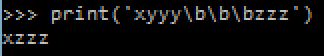Separate prints

We also probably want to use separate prints rather than put everything in the same print statement. To do this you need to make sure that the print will not start a new line at the end. In Python 3 you can add the argument "end=''" to the print:
def f():
print('xy', end='')
print('\bz')

f()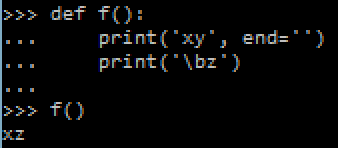def f():
print('xy', end='')
print('\rzz')

f()Alternatively you can use the sys.stdout.write function which is equivalent to print without the extra features such as adding a new line at the end:
import sys

def f():
sys.stdout.write('xy')
sys.stdout.write('\bz\n')

f()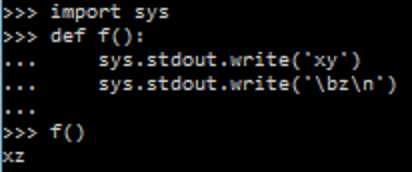String formatting

When displaying a percentage it's important that the number always takes the same amount of character space so that you know how many characters to overwrite. This can be done using the format method of strings. The format method allows you to make a string take a fixed amount of characters, filling the remainder with spaces as follows:
curr = 12
total = 21
frac = curr/total
print('[{:>7.2%}]'.format(frac))The formatting code is the "{:>7.2%}", where ">" means that the string is be right aligned with spaces added to the front, "7" is the fixed length of the string + padded spaces (3 whole number digits + a point + 2 decimal digits + a percentage sign) ".2" is the number of decimal places to round the percentage to, and "%" means that the fraction should be expressed as a percentage.

Replicated strings

When displaying a progress bar it would also be useful to know that you can replicate a string for a number of times using the '*' operator, as follows:
curr = 12
total = 21
full_progbar = 10
filled_progbar = round(curr/total*full_progbar)
print('#'*filled_progbar + '-'*(full_progbar-filled_progbar))Full example

Here is a full progress bar example:

def progbar(curr, total, full_progbar):
frac = curr/total
filled_progbar = round(frac*full_progbar)
print('\r', '#'*filled_progbar + '-'*(full_progbar-filled_progbar), '[{:>7.2%}]'.format(frac), end='')

for i in range(100000+1):
progbar(i, 100000, 20)
print()It might also be the case that the progress bar is not updating consistently and seems to be getting stuck. This could be because the print is buffering and need to be "flushed" after every print. This is done by adding
sys.stdout.flush()

after every print (don't forget to import "sys" before).

## Tuesday, June 21, 2016

### The Backpropagation Algorithm for Artificial Neural Networks (ANNs)

In a previous post, I talked about how to use the gradient descent algorithm to optimize the weights and biases of an artificial neural network in order to give selected outputs for selected inputs. However plain gradient descent is inefficient on large neural nets since it recomputes a lot of values for each neuron, plus it has to be rewritten for every change in architecture (number of neurons and layers) and requires a lot of code. The standard solution is to use an optimized version of the gradient descent algorithm for neural nets called the backpropagation algorithm.

I will assume that you have read the previous post. Whereas the previous post was very specific using a specified architecture and a specified activation and cost function, here I will keep things as general as possible such that they can be applied on any feed forward neural network. Here are the definitions of symbols we shall be using, similar to last post's definitions:In order to help explain the algorithm and equations, I shall be applying it to the last post's architecture, just to help you understand. So here is last post's neural net: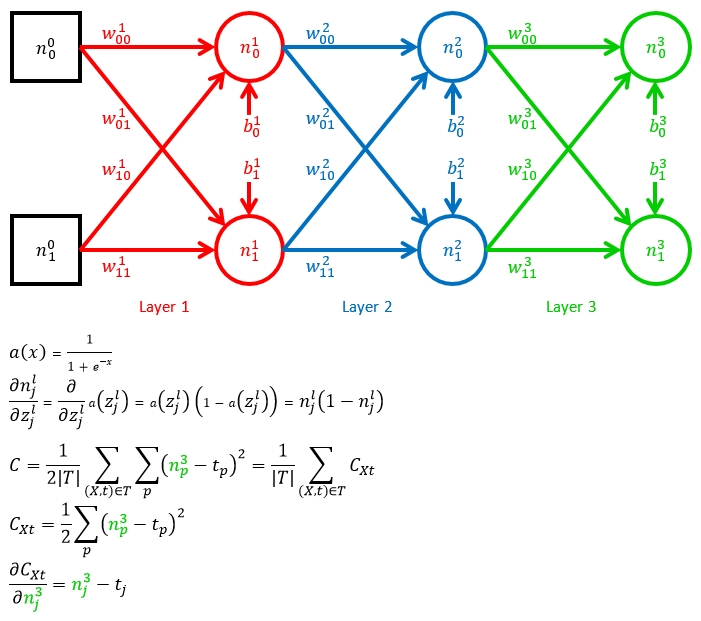In the example, we have a 3 layer neural net with 2 neurons in each layer and 2 input neurons. It uses the sigmoid function as an activation function and the mean square error as the cost function.

The main point of interest in the backpropagation algorithm is the way you find the derivative of the cost function with respect to a weight or bias in any layer. Let's look at how to find these derivatives for each layer in the example neural net.

Weights and bias in layer L (output layer)

We begin by finding the derivatives for the output layer, which are the simplest.

d Cost / d Weight L
The derivative of the cost with respect to any weight in layer L can be found using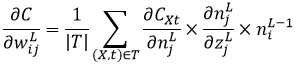Here's how we can arrive to this equation based on a weight in layer 3 in the example: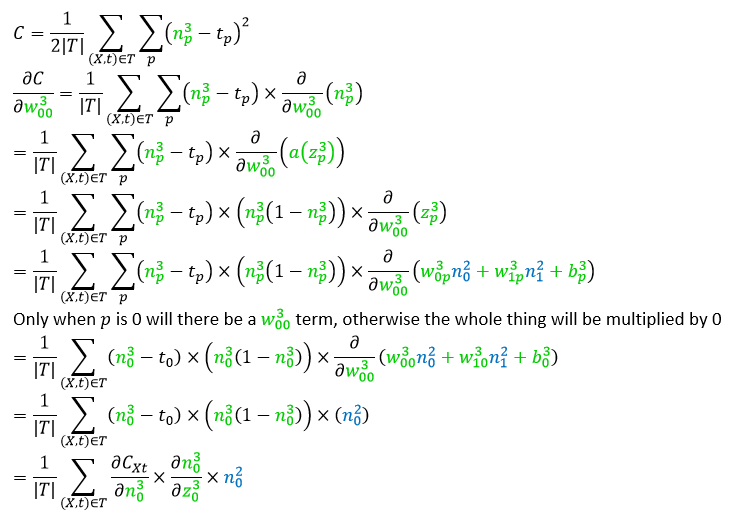d Cost / d Bias L

The derivative of the cost with respect to any bias in layer L can be found using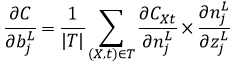Here's how we can arrive to this equation based on a bias in layer 3 in the example:Using delta

A part of the weights and biases equations is repeated. It will be convenient when finding derivatives of other layers to use an intermediate letter, called lower case delta, to represent this part of these equations.Weights and bias in layer L-1

Now we find the derivative with respect to the weights and biases in layer L-1. The derivations will be continuations of the previous derivations and we won't be repeating the first bit of the derivations again.

d Cost / d Weight L-1
The derivative of the cost with respect to any weight in layer L-1 can be found using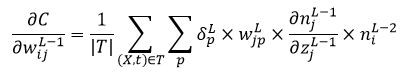Here's how we can arrive to this equation based on a weight in layer 2 in the example:d Cost / d Bias L-1

The derivative of the cost with respect to any bias in layer L-1 can be found using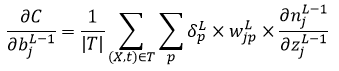Here's how we can arrive to this equation based on a bias in layer 2 in the example: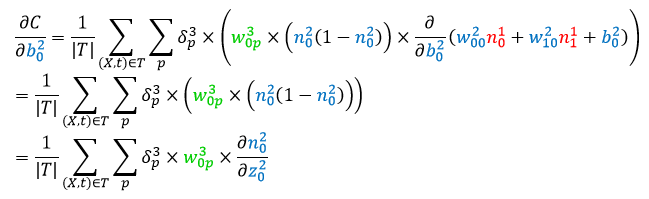Using delta

These equations can be shortened by using delta again and now we can use the previous delta to shorten this one even more.Notice how there is a recursive definition of delta. This is the basis for the backpropagation algorithm.

Weights and bias in layer L-2

Now we find the derivative with respect to the weights and biases in layer L-2. Like before, we won't be repeating the first bit of the derivations again.

d Cost / d Weight L-2
The derivative of the cost with respect to any weight in layer L-2 can be found using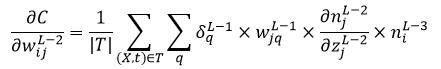Here's how we can arrive to this equation based on a weight in layer 1 in the example (notice that there is a trick we'll be using with the summations that is explained below):At the end of the derivation we did a trick with the sigmas (summations) in order to turn the equation into a recursive one.

First, we moved the delta to the inside of the sigmas. This can be done because it's just a common term that is factored out and we're factoring it back in. For example if the equation is "𝛿(a + b + c)" then we can turn that into "𝛿a + 𝛿b + 𝛿c".

Second, we swapped the "p" summation with the "q" summation. This does not change anything if you think about it. All it does is change the order of the summations, which does not change anything.

Finally we replaced the "p" summation with the previous delta, allowing us to shorten the equation.

d Cost / d Bias L-2

The derivative of the cost with respect to any bias in layer L-2 can be found usingHere's how we can arrive to this equation based on a bias in layer 1 in the example:Using delta

Again, we can shorten the equations using delta.Notice that this is exactly the same as the previous delta. This is because beyond L-1, all the deltas will be identical and can be defined using a single recursive relation.

In general: using indices

Up to now we saw what the derivatives for layer L, L-1, and L-2 are. We can now generalize these equations for any layer. Given the recursive pattern in the deltas, we can create an equation that gives the deltas of any layer provided you have the deltas of the previous layers. The base case of the recursion would be for layer L, which is defined on its own.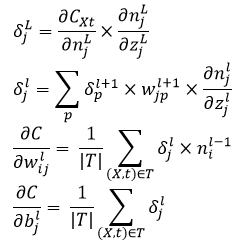Now we have a way to find the derivatives for any layer, no matter how deep the neural network is. Here is how you'd use these equations on the example: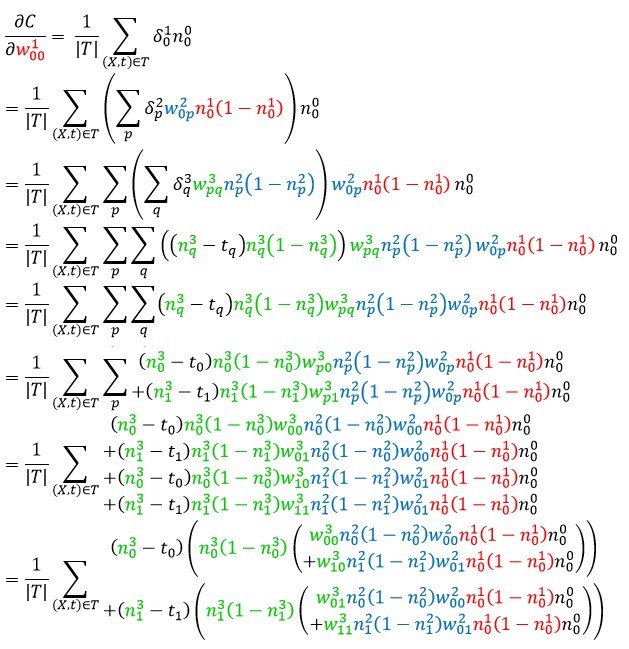In general: using matrices

As things stand, there are a lot of index letters littering our equations (i,j,p). We can get rid of them however if we use matrix operations that work on all the indices at once. If we do this we can even get shorter code when programming the backpropagation algorithm by making use of a fast matrix library such as numpy.

Using matrices in neural nets

Let's look at how we can use matrices to compute the output of a neural net. A whole layer of activations can be calculated as a whole by treating it as a vector. We'll treat vectors as being horizontal by default and which need to be transposed in order to be made vertical.

Here's an example of what we want to calculate: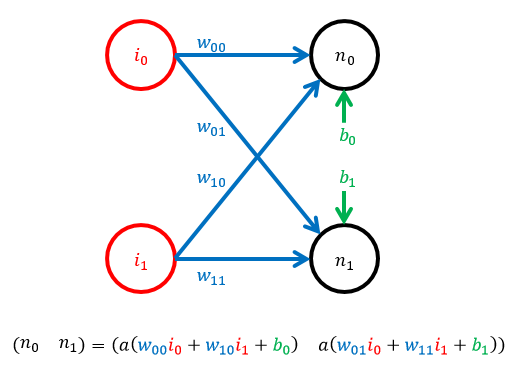Of course we're still using indices just because we're organising our activations in a vector. In order to get rid of the indices we need to treat the weights as a matrix, where the first index is the row number and the second index is the column number. That way we can have a single letter "w" which represents all of the weights of a layer. When multiplied by a vector of the previous layer's activations, the matrix multiplication will result in another vector of weighted sums which can be added to a bias vector and passed through an activation function to compute the next vector of activations. Here is an example of the calculation based on the above example:Final clean generalized deltas and cost gradients

And now we can finally see what the clean matrix-based general derivatives are for any layer: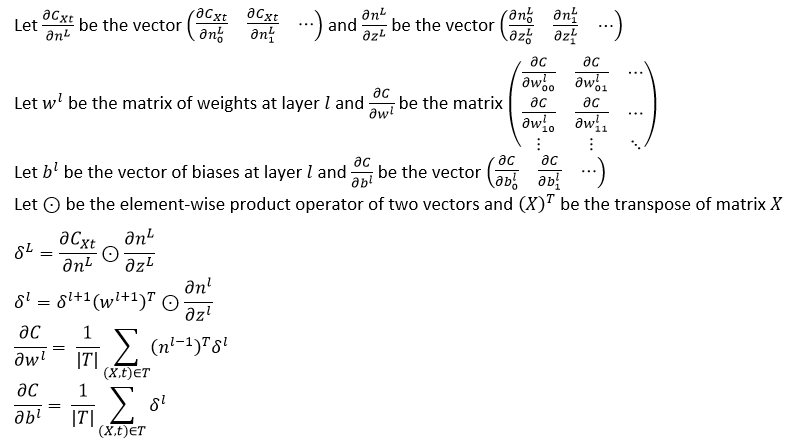The backpropagation algorithm

Finding the gradients is one step (the most complex one) of the backpropagation algorithm. The full backpropagation algorithm goes as follows:

1. For each input-target pair in the training set,
1. Compute the activations and the "z" of each layer when passing the input through the network. This is called a forward pass.
2. Use the values collected from the forward pass to calculate the gradient of the cost with respect to each weight and bias, starting from the output layer and using the delta equations to compute the gradients for previous layers. This is called the backward pass.
2. Following the backward pass, you have the gradient of the cost with respect to each weight and bias for each input in the training set. Add up all the corresponding gradients of each input in the training set (all the gradients with respect to the same weight or bias).
3. Now use the gradient to perform gradient descent by multiplying the gradient by a step size, or learning rate as it's called in the backpropagation algorithm, and subtracting it from the corresponding weights and biases. In algebra,
weight = weight - learningrate*dCost/dWeight

In code
And this is what the full program looks like in Python 3 using numpy to perform matrix operations. Again, we shall be training the neural net to perform the function of a half adder. A half adder adds together two binary digits and returns the sum and carry. So 0 + 1 in binary gives 1 carry 0 whilst 1 + 1 in binary gives 0 carry 1.

import math, random
import numpy as np

class ANN:
'''General artificial neural network class.'''

def __init__(self, num_in, num_hiddens, num_out, trainingset):
'''Create a neural network with a set number of layers and neurons and with initialised weights and biases.'''
if not all(len(x)==num_in and len(y)==num_out for (x,y) in trainingset.items()):
raise ValueError()

self._num_in = num_in
self._num_hiddens = num_hiddens
self._num_out = num_out
self._trainingset = trainingset

self._num_layers = len(num_hiddens) + 1

self._weights = dict([
(1, np.array([
[self.weight_init(1, i, j) for j in range(num_hiddens)]
for i in range(num_in)
]))
]
+ [
(l+1, np.array([
[self.weight_init(l+1, i, j) for j in range(num_hiddens[l])]
for i in range(num_hiddens[l-1])
]))
for l in range(1, len(num_hiddens))
]
+ [
(self._num_layers, np.array([
[self.weight_init(self._num_layers, i, j) for j in range(num_out)]
for i in range(num_hiddens[-1])
]))
])

self._biases = dict([
(l+1, np.array([
self.bias_init(l+1, j) for j in range(num_hiddens[l])
]))
for l in range(len(num_hiddens))
]
+ [
(self._num_layers, np.array([
self.bias_init(self._num_layers, j) for j in range(num_out)
]))
])

def weight_init(self, layer, i, j):
'''How to initialise weights.'''
return 2*random.random()-1

def bias_init(self, layer, j):
'''How to initialise biases.'''
return 2*random.random()-1

def a(self, z):
'''The activation function.'''
return 1/(1 + np.vectorize(np.exp)(-z))

def da_dz(self, z):
'''The derivative of the activation function.'''
return self.a(z)*(1-self.a(z))

def out(self, x):
'''Compute the output of the neural network given an input vector.'''
n = x
for l in range(1, self._num_layers+1):
n = self.a(np.dot(n, self._weights[l]) + self._biases[l])
return n

def cost(self):
'''The cost function based on the training set.'''
return sum(sum((self.out(x) - t)**2) for (x,t) in self._trainingset.items())/(2*len(self._trainingset))

def dCxt_dnL(self, x, t):
'''The derivative of the cost function for a particular input-target pair with respect to the output of the network.'''
return self.out(x) - t

'''Calculate and return the gradient of the cost function with respect to each weight and bias in the network.'''
dC_dws = dict()
dC_dbs = dict()
for l in range(1, self._num_layers+1):
dC_dws[l] = np.zeros_like(self._weights[l])
dC_dbs[l] = np.zeros_like(self._biases[l])

for (x,t) in self._trainingset.items():
#forward pass
zs = dict()
ns = dict()
ns_T = dict()
ns = np.array(x)
ns_T = np.array([np.array(x)]).T
for l in range(1, self._num_layers+1):
z = np.dot(ns[l-1], self._weights[l]) + self._biases[l]
zs[l] = z
n = self.a(z)
ns[l] = n
ns_T[l] = np.array([n]).T

#backward pass
d = self.dCxt_dnL(x, t)*self.da_dz(zs[self._num_layers])
dC_dws[self._num_layers] += np.dot(ns_T[self._num_layers-1], np.array([d]))
dC_dbs[self._num_layers] += d
for l in range(self._num_layers-1, 1-1, -1):
d = np.dot(d, self._weights[l+1].T)*self.da_dz(zs[l])
dC_dws[l] += np.dot(ns_T[l-1], np.array([d]))
dC_dbs[l] += d

for l in range(1, self._num_layers+1):
dC_dws[l] /= len(self._trainingset)
dC_dbs[l] /= len(self._trainingset)

return (dC_dws, dC_dbs)

def epoch_train(self, learning_rate):
'''Train the neural network for one epoch.'''
for l in range(1, self._num_layers+1):
self._weights[l] -= learning_rate*dC_dws[l]
self._biases[l] -= learning_rate*dC_dbs[l]

'''Check if the gradients are being calculated correctly. This is done according to http://ufldl.stanford.edu/tutorial/supervised/DebuggingGradientChecking/ . This method calculates the difference between each calculated gradient (according to get_cost_gradients()) and an estimated gradient using a small number epsilon. If the gradients are calculated correctly then the returned numbers should all be very small (smaller than 1e-10.'''

approx_dC_dws = dict()
approx_dC_dbs = dict()
for l in range(1, self._num_layers+1):
approx_dC_dws[l] = np.zeros_like(self._weights[l])
approx_dC_dbs[l] = np.zeros_like(self._biases[l])

for l in range(1, self._num_layers+1):
(rows, cols) = self._weights[l].shape
for r in range(rows):
for c in range(cols):
orig = self._weights[l][r][c]
self._weights[l][r][c] = orig + epsilon
cost_plus = self.cost()
self._weights[l][r][c] = orig - epsilon
cost_minus = self.cost()
self._weights[l][r][c] = orig
approx_dC_dws[l][r][c] = (cost_plus - cost_minus)/(2*epsilon)

(cols,) = self._biases[l].shape
for c in range(cols):
orig = self._biases[l][c]
self._biases[l][c] = orig + epsilon
cost_plus = self.cost()
self._biases[l][c] = orig - epsilon
cost_minus = self.cost()
self._biases[l][c] = orig
approx_dC_dbs[l][c] = (cost_plus - cost_minus)/(2*epsilon)

errors_w = dict()
errors_b = dict()
for l in range(1, self._num_layers+1):
errors_w[l] = abs(predicted_dC_dws[l] - approx_dC_dws[l])
errors_b[l] = abs(predicted_dC_dbs[l] - approx_dC_dbs[l])
return (errors_w, errors_b)

################################################################
nn = ANN(
2, #number of inputs
[ 2, 2 ], #number of hidden neurons (each number is a hidden layer)
2, #number of outputs
{ #training set
(0,0):(0,0),
(0,1):(1,0),
(1,0):(1,0),
(1,1):(0,1)
}
)

print('Initial cost:', nn.cost())
print('Starting training')
for epoch in range(1, 20000+1):
nn.epoch_train(0.5)
if epoch%1000 == 0:
print(' Epoch:', epoch, ', Current cost:', nn.cost())
print('Training finished')

print('Neural net output:')
for (x,t) in sorted(nn._trainingset.items()):
print(' ', x, ':', nn.out(x))


After 20,000 iterations I got this output:
Initial cost: 0.232581149941
Starting training
Epoch: 1000 , Current cost: 0.218450291043
Epoch: 2000 , Current cost: 0.215777328824
Epoch: 3000 , Current cost: 0.178593050179
Epoch: 4000 , Current cost: 0.102704613785
Epoch: 5000 , Current cost: 0.0268721186425
Epoch: 6000 , Current cost: 0.00819827730488
Epoch: 7000 , Current cost: 0.00444660869981
Epoch: 8000 , Current cost: 0.00298786209459
Epoch: 9000 , Current cost: 0.00223137023455
Epoch: 10000 , Current cost: 0.00177306322414
Epoch: 11000 , Current cost: 0.00146724847349
Epoch: 12000 , Current cost: 0.00124934223594
Epoch: 13000 , Current cost: 0.00108652952015
Epoch: 14000 , Current cost: 0.000960438140866
Epoch: 15000 , Current cost: 0.000860007442299
Epoch: 16000 , Current cost: 0.000778192177283
Epoch: 17000 , Current cost: 0.000710297773182
Epoch: 18000 , Current cost: 0.000653078479503
Epoch: 19000 , Current cost: 0.000604220195232
Epoch: 20000 , Current cost: 0.0005620295804
Training finished
Neural net output:
(0, 0) : [ 0.02792593  0.00058427]
(0, 1) : [ 0.97284142  0.01665468]
(1, 0) : [ 0.97352071  0.01661805]
(1, 1) : [ 0.03061993  0.97196112]


## Sunday, May 22, 2016

### Making your own deep learning workstation: From Windows to Ubuntu dual boot with CUDA and Theano

I recently managed to turn my Windows 7 gaming PC into a dual boot GPU enabled deep learning workstation which I use for deep learning experiments. Here is how I did it.

First of all, you'll want to use Theano as a deep learning framework which automatically optimises your code to use the GPU, the fast parallel processor on your graphics card, which makes deep learning faster (twice as fast on my computer). Unfortunately this seems to be difficult to do on Windows, but easy to do on Linux; so I created a partition for Ubuntu Linux (splitting a hard disk into two separate spaces which can contain two different operating systems) and made my computer dual boot (so that I can choose whether to use Windows or Ubuntu when my computer is switched on). It seems that the most compatible Ubuntu version which most tutorials focus on is Ubuntu 14.04, which is what I installed. I then installed CUDA, which is the NVIDIA graphic card driver that allows you to write programs that use the GPU. After installing this, I installed Theano together with the even easier specialisation framework Keras which lets you do deep learning with only a few lines of Python code.

Be warned that I had to reinstall Ubuntu multiple times because I followed different tutorials which didn't work. Here I will link to the tutorials which worked for me.

Create a partition for Ubuntu
Tutorial
The first step is to create a partition in your C: drive in which to install Ubuntu. I made a partition of 100GB using the Windows Disk Management tool. Follow the instructions in the tutorial.

Install Ubuntu 14.04 into the partition (yes, it is recommended to use 14.04), together with making the computer dual boot
Tutorial
Follow the instructions in the tutorial to use BCDEdit, the Windows boot manager tool, to make it possible to choose whether to use Windows or Ubuntu. Stop at step 5.

At this point you're on step 6 of the tutorial. Be sure to choose "something else" when asked how to install Ubuntu (whether it's along side your other operating system or remove whatever you already have in your computer). Create a 1GB partition for swap space and another partition with the remainder for the available unformatted space for Ubuntu. You can find instructions on this at http://askubuntu.com/questions/343268/how-to-use-manual-partitioning-during-installation/521195#521195. It's very important that the device for boot loader is set to be the same partition where Ubuntu will be installed, otherwise Windows will not show when you turn on your computer. Do not restart, but choose to continue testing the Live CD instead.

You are now in step 8 in the tutorial. This is where you make it possible to select Ubuntu when you switch on your computer. Here is a list of steps you need:
1. First, you need to mount the Windows and Ubuntu partitions to make them accessible. In Ubuntu there is no "My Computer" like in Windows where you can see all the partitions. Instead you have the drives and partitions shown on the side. Click on the ones whose size matches those of the Windows C: drive and the new Ubuntu partition (after clicking on them they will be opened so you can check their contents). This will to mount the partitions.
2. Next you need to know the partition name of the Ubuntu partition. Click on the Ubuntu icon on the side and search for a program called GParted. Open the program and use the drop down list in the top corner to look for the partition you created for Ubuntu (selecting different choices in the drop down list will show you partitions in different physical hard disks you have). Take note of the name of the Ubuntu partition such as "/dev/sda2". Call this name [Ubuntu partition].
3. Next you need to know the directory path to the Windows partition. In an open folder window (or go on the Files icon in the side), click on "Computer" on the side, go in "media", enter into the "ubuntu" folder and there you will find all the mounted partitions. Find the Windows partition. Call the directory path to this partition icon [Windows partition] (/media/ubuntu/abcdef-123456).
4. Open the terminal (ctrl+alt+T) and then just copy the following line into the terminal. Where it says '[Windows parition]' just drag and drop the partition icon into the terminal and the whole directory will be written for you.
sudo dd if=[Ubuntu partition] of='[Windows partition]/ubuntu.bin' bs=512 count=1

Now you can restart, remove the DVD, and log into your newly installed Ubuntu operating system, update it, and do another restart.

Installing CUDA
Tutorial
This is the part that took the most tries since only one tutorial actually worked well and gave no errors. Follow the instructions in the tutorial. When downloading the CUDA driver be sure to download the .deb file.

Installing Theano with dependencies
Tutorial
Now comes the straight forward part. Unfortunately it's easier to just use Python 2 than to try to make things work with Python 3. The examples in Keras are for Python 2 anyway. Just open the terminal and paste:
sudo apt-get install python-numpy python-scipy python-dev python-pip python-nose g++ libopenblas-dev git

However also install an extra package which will let you test that Theano was installed correctly:
sudo pip install nose-parameterized

Now you can install theano and keras.
sudo pip install Theano
sudo pip install keras


Now whenever you want to run a theano or keras python script and use the GPU all you have to do is write the following in the terminal:
THEANO_FLAGS=mode=FAST_RUN,device=gpu,floatX=float32 python [python script]

You may also want to use Python IDLE in order to experiment with theano, in which case install it like this:
sudo apt-get install idle
and then run it to use the GPU like this:
THEANO_FLAGS=mode=FAST_RUN,device=gpu,floatX=float32 idle
or do this to debug an error in your program:
THEANO_FLAGS=mode=FAST_COMPILE,device=cpu,floatX=float32 idle

I also recommend this good theano tutorial to get you started.

## Sunday, April 17, 2016

### The Kullback–Leibler divergence

In a text file, each character is represented by a number of bits. Usually each character consists of 8 bits. But in data compression and information theory we learn that the number of bits need not be the same for each character. The most frequent characters can be given the fewest number of bits whilst the least frequent characters can be given many bits. According to Shannon's information theory, the smallest number of bits needed to represent a character that occurs with probability "p" is
-log2(p)

This means that if a character occurs half the time in a document, then you need -log2(0.5) bits which equals 1. On the other hand a character that occurs an eighth of the time should be represented with -log2(0.125) bits which equals 3. The rarer the character, the more bits should be assigned to it in order to be able to use less bits for the more frequent characters. This will result in compressing the whole document.

The entropy of a document is the expected number of bits per character, that is, the average number of bits in a document. If the number of bits is minimum, as described above, the expected number of bits is
-sum(p log2(p) for all character probabilities p)

Now that we know what entropy is, we can understand what the Kullback-Leibler divergence is. Assume that the number of bits for each character is calculated based on a different document than the one being compressed. Clearly this is a recipe for disaster, but you might want to compute an average probability for each character once based on a representative corpus and then always use these probabilities in all documents, which saves time. By how much will the probabilities diverge? This is what the Kullback-Leibler divergence is used for.

What we'll do is we'll calculate the difference in the average number of bits per character when using the wrong probabilities instead of the correct ones. This is done as follows:
-sum(p log2(q)) - -sum(p log2(p))


Notice how the correct probability for a particular character is "p" but in the first term we're using a different probability "q". This can now be simplified as follows:
-sum(p log2(q)) - -sum(p log2(p))
sum(p log2(p)) - sum(p log2(q))
sum(p log2(p) - p log2(q))
sum(p(log2(p) - log2(q)))
sum(p log2(p/q))


And this is the Kullback-Leibler divergence between the probabilities that "p" comes from and the probabilities that "q" comes from. It is usually used to measure the difference between two probability distributions.

## Monday, March 14, 2016

Happy pi day. Here's how you can display as many digits of pi as you want (subject to your computer's resources).

We're going to be using Gibbon's unbounded spigot algorithm which spits out consecutive digits of pi in decimal without iterative approximations. It's really cool when you see it in action.

In the above linked paper, the algorithm is given in Haskell. I won't explain the mathematical derivation in this post, but here is a translation into Python 3:
def gibbons():
(q, r, t, k, n, l) = (1, 0, 1, 1, 3, 3)
while True:
if 4*q + r - t < n*t:
yield n
(q, r, n) = (
10*q,
10*(r - n*t),
10*(3*q + r)//t - 10*n
)
else:
(q, r, t, k, n, l) = (
q*k,
(2*q + r)*l,
t*l,
k+1,
(q*(7*k + 2) + r*l)//(t*l),
l + 2
)

To use this code run this:
digit_seq = gibbons()
print(next(digit_seq))
print(next(digit_seq))
print(next(digit_seq))
print(next(digit_seq))

This will output
3
1
4
1

If you want it to print out a thousand digits, do this:
digit_seq = gibbons()
for (position, digit) in enumerate(digit_seq):
if position == 1000:
break
print(digit, end='')

Now this code will let you print as many digits as you want; however being in Python it's not something you can just tell your non-geek friend to try out. So for pi day, here's a javascript implementation that anyone can just paste in their browser's web console and get a nice stream of pi digits. One thing you should keep in mind is that in Python you can have numbers which are as big as you like. Javascript is not like that, so we'll need to use an external library called bignumber.js. This is the full code we'll be using to generate the digits:
var script = document.createElement('script');
script.src = "https://cdnjs.cloudflare.com/ajax/libs/bignumber.js/2.3.0/bignumber.min.js";

function gibbons() {
var q=new BigNumber(1), r=new BigNumber(0), t=new BigNumber(1), k=new BigNumber(1), n=new BigNumber(3), l=new BigNumber(3);
function f() {
while (true) {
var digit = n.toString();
var q_ = q.mul(10);
var r_ = r.sub(n.mul(t)).mul(10);
q=q_, r=r_, n=n_;
return digit;
}
else {
var q_ = q.mul(k);
var t_ = t.mul(l);
q=q_, r=r_, t=t_, k=k_, n=n_, l=l_;
}
}
}
return f;
}

function show_digits(max_num_digits) {
var get_digit = gibbons();
var num_digits = 0;
function f() {
var digits = '';
for (var i = 1; i <= 50; i++) {
digits += get_digit();
num_digits++;
if (num_digits >= max_num_digits) {
break;
}
}
console.log(digits);
if (num_digits < max_num_digits) {
setTimeout(f, 100);
}
else {
console.log('\n'+max_num_digits+' digits of pi displayed!');
}
}
f();
}

show_digits(1000);

The first line is to import the bignumber library in a way that works in the web console. After that there are two functions. The first is for the Gibbons algorithm which lets us get the digits of pi. The way it works is by returning a function which returns the next digit each time it is called. The second function outputs 50 digits of pi every 100 milliseconds.
The code to copy-paste
But this is not something you'll send you friend. Instead send them this minified code (of course you shouldn't trust obscure code sent by strangers as it might be evil code, but you can minify the above code youself if you don't trust me).
var script=document.createElement("script");script.src="https://cdnjs.cloudflare.com/ajax/libs/bignumber.js/2.3.0/bignumber.min.js",document.getElementsByTagName("head").appendChild(script);

show_digits(1000);


What you have to do is open your browser's web console on any web page by pressing F12 (even a blank tab works, although Facebook will block it), then paste the first line of code at the bottom. After pressing enter, paste the second line of code. Finally paste the "show_digits(1000);" line and press enter. You'll see a stream of digits displayed in the console panel. I think this is a nice way to celebrate pi day. Maybe someone else can make the digits somehow interact with the web page you are on instead of just display them in the console. Until then, enjoy your pie today!

## Sunday, February 28, 2016

### Gradient Descent Algorithm on Artificial Neural Networks (ANNs)

Polynomials are great. They're a simple mathematical equation pattern which is simple and easy to work with. You basically have a number of constants "a", "b", "c", etc. which you use in the following pattern:
f(x) = a x^0 + b x^1 + c x^2 + ...

By changing the values of the constants you change the shape of the graph of f(x). You can design a particular graph shape by choosing the values of these constants. In fact, one of the most interesting properties of polynomials is that they can be easily designed so that their graph passes exactly through a given set of points. For example, you can choose the constants of the polynomial to make f(1) = 2, f(3) = -1, f(-1.5) = 0.5, etc. This is very easy to make using Lagrange polynomials. The constants of the polynomial can be seen as parameters which can be optimized in order to get a desired function.

Polynomials are not the only equation which is designed to be easily modifiable. One of the most well known modifiable equation patterns are Artificial Neural Networks. Although neural networks are inspired by the neurons in the brain and how they change when learning to perform new tasks, the concept behind neural networks is that of having an equation that, by design, can be easily modified to turn chosen inputs into chosen outputs. They can take any number of inputs and outputs and they usually only work on binary numbers (which is great for computers). They have been used for lots of things such as recognising objects in images and classifying text into meaningful categories. Neural networks are known to generalise a set of input-output mappings into useful functions that work well for new inputs. For example, if you design a neural network to map 100 particular pictures of dogs to the output "1" and another 100 particular pictures of cats to the output "0", then the network will start giving the expected output for new pictures of dogs and cats.

Neural network definition

Whereas the building block of the polynomial is the term "k x^i", the building block of the neural network is the neuron. A neuron takes in a number of signals from other neurons, weighs the signals by their importance by making them weaker or stronger, adds them together and if the sum is over a particular threshold, then the neuron will output its own signal to some other neurons. Here is a diagram of this:Neurons are organized into layers, where one layer of neurons supplies input signals to the next layer of neurons. In mathematical terms, a neuron is defined as follows:Activation functions are functions that sharply change value when the input is over a certain threshold. Traditionally, neural networks use the sigmoid function given above which has a graph like this:See how the value changes from 0 to 1 after passing x = 0? This is what will make the neuron output a signal if the weighted sum is over a threshold. Left as is, the threshold is 0; but we can change the threshold by changing the value of the bias in order to shift the graph to the left or right. The weighting of input signals by importance is done by multiplying the input by a weight, which can be a large number, a small fraction, or even a negative number.

Just like you can modify polynomial functions by changing their constants, you can modify neural network functions by changing their weights and biases. Further down we'll see how to do this.

Neural network example

Given the above definitions, here is a diagram of an example of a neural network: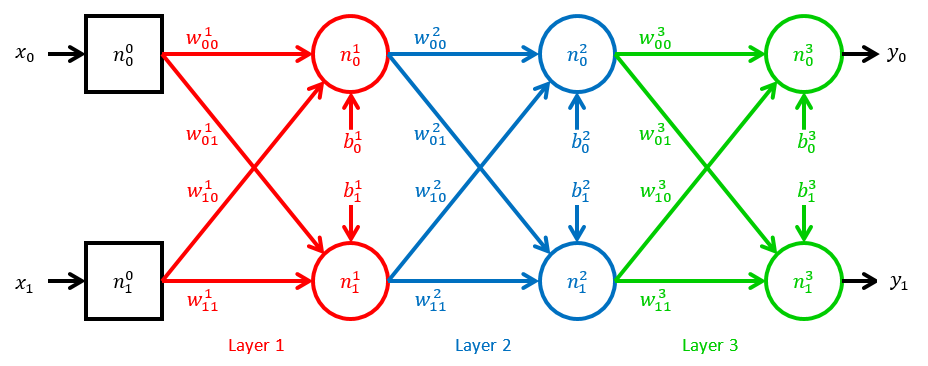We shall be referring to this diagram throughout this blog post. Notice the following things about it:
• Its 2 inputs are called "x0" and "x1" whilst its 2 outputs are called "y0" and "y1".
• Since there are two outputs, the output of the whole network is a column vector of two numbers.
• The first layer of neurons are square because they propagate the "x" signals to the next layer unmodified. The rest of the neurons work as explained.
• Each circle neuron receives input signals from all neurons in the previous layer.
• Apart from input signals, each circle neuron also accepts a bias signal that is always the same.

This diagram is just a visual representation of the equation shown below. The equation is derived layer by layer from the output. Notice how the equation is a column vector of two numbers since there are two outputs.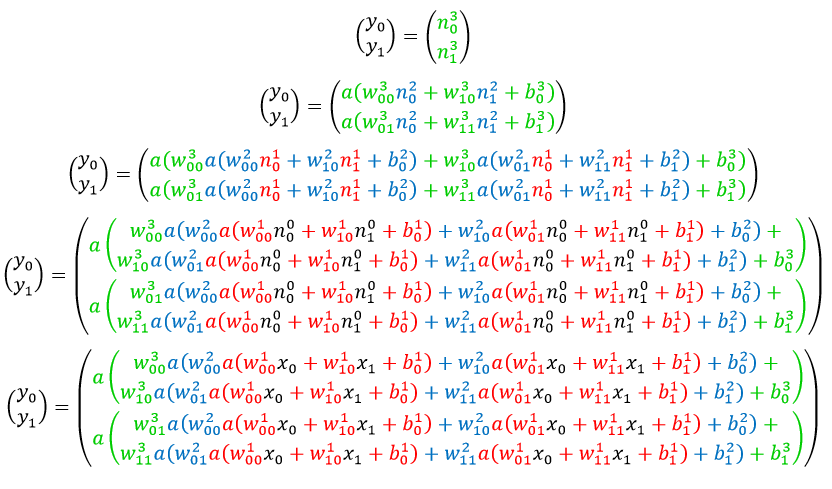Notice that we are representing the neural network using normal algebra instead of using linear algebra with matrices as usual. I believe that this makes the example easier to understand. Linear algebra is useful for generalising the concepts to any neural network shape, but for understand one network example we'll stick to this expanded representation.

Neural network modification

So now we know how to create a neural network equation, but how do we choose its weights and biases in order to get the function we want? In the previous blog post, we talked about how to use the gradient descent algorithm in order to numerically find the minimum of an equation when solving the equation algebraically is difficult. In fact, one of the most well known uses of the gradient descent algorithm is for "training" neural networks into performing a desired function, that is, giving desired outputs from particular inputs.

Usually neural networks are trained using the backpropagation algorithm which is based on the gradient descent algorithm. However we'll see how to do the training using plain gradient descent which will help us understand the backpropagation algorithm in a later blog post.

What we'll do is create a "cost" function that quantifies how close the outputs that the network is giving are to the desired outputs. Suppose that we want the above neural network to output (0,1) when given (0,0) and (1,0) when given (1,1). The cost function will measure how far off the neural network is from actually giving these outputs. If the cost function gives 0, then the network is giving exactly these desired outputs, otherwise it will be larger than zero. Different weights and biases will make the cost function give different values and our job is to find the combination of weights and biases that will give a cost of zero. We will do this using the gradient descent algorithm.

There are many possible cost functions, but traditionally, this is how we define the cost function: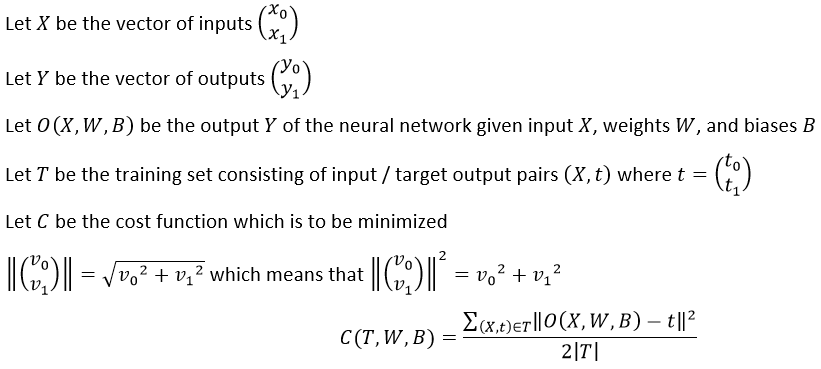Notice that O(X,W,B) = Y.

The cost function here is measuring how far the actual output is from the target output by calculating the mean squared error. For convenience later on we're also dividing the equation by 2.

For the above network, the cost function applies as follows:Now that we have a continuous equation that quantifies how far from the desired function our neural network's weights and biases are, we can use gradient descent in order to optimize the weights and biases into giving the desired function. To do that we need to find the partial derivative of the network's equation with respect to every weight and bias. Before we do so, notice that the derivative of the activation function is as follows:The above equation is saying that the derivative of a neuron's output is defined using the neuron's output (the output multiplied by one minus the output). This is a useful speed optimization in sigmoid activation functions.

We shall now find the partial derivative of two weights and two biases. You can then find the partial derivatives of every other weight and bias yourself. Make sure that you know how the chain rule in differentiation works. As you follow the derivations, keep in mind that all the "y"s and "n"s are actually functions of "B", "W", and "X" just like "O", but we'll leave the "(X,W,B)" out in order to save space.

Partial derivative with respect to weight in layer 3 (output layer)

Let's find the partial derivative of one of the weights in layer 3 (output layer).Notice how the 2 in the denominator was cancelled with the 2s in the numerator. This is why we put it in the denominator of the cost function. Notice also that the summation does not change anything in the derivative since the derivative of a summation is the summation of the summed terms' derivative.

Now we'll find the derivative of each "y" separately.Notice that we stopped decomposing neurons past layer 3 since we know that the weight we are deriving with respect to will not lie in deeper layers.

Finally we plug these back into the original equation and we have our partial derivative:It's important to notice that the derivative uses the output of the network's neurons. This is important, since it means that before finding the derivative we need to first find the output of the network. Notice also that the green "n" at layer 3 is "y0" but we won't replace it with "y0" in order to preserve a pattern that is revealed later.

Partial derivative with respect to bias in layer 3 (output layer)

Let's find the partial derivative of one of the biases in layer 3 (output layer) now. We'll skip some steps that were shown in the previous derivations.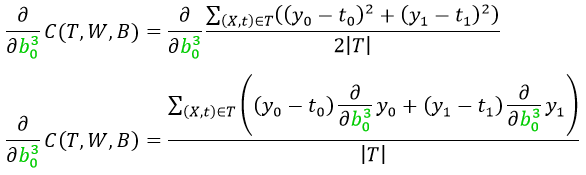And now we find the derivatives of the "y"s separately.And we now plug them back into the original equation.Partial derivative with respect to weight in layer 2

Let's find the partial derivative of one of the weights in layer 2 now. We'll skip some steps that were shown in the previous derivations.And now we find the derivatives of the "y"s separately.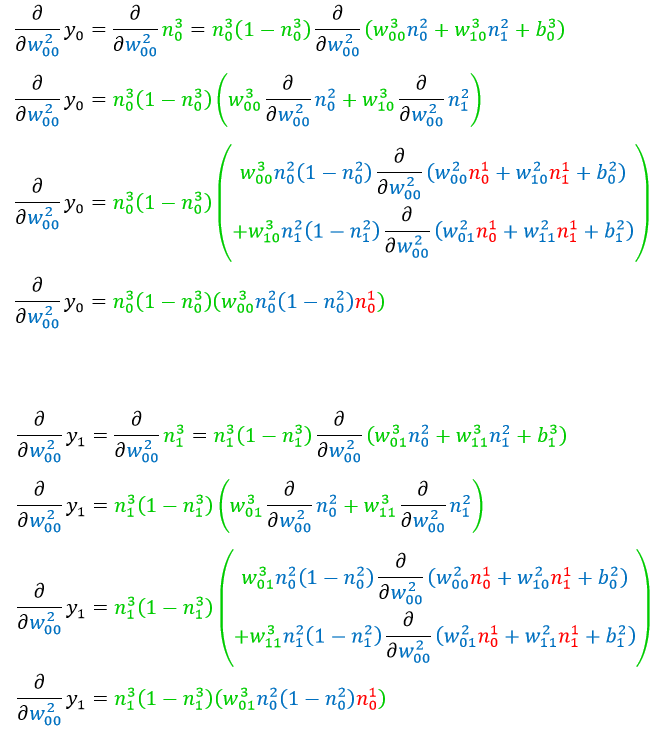And we now plug them back into the original equation.Partial derivative with respect to bias in layer 2

Let's find the partial derivative of one of the biases in layer 2 now. We'll skip some steps that were shown in the previous derivations.And now we find the derivatives of the "y"s separately.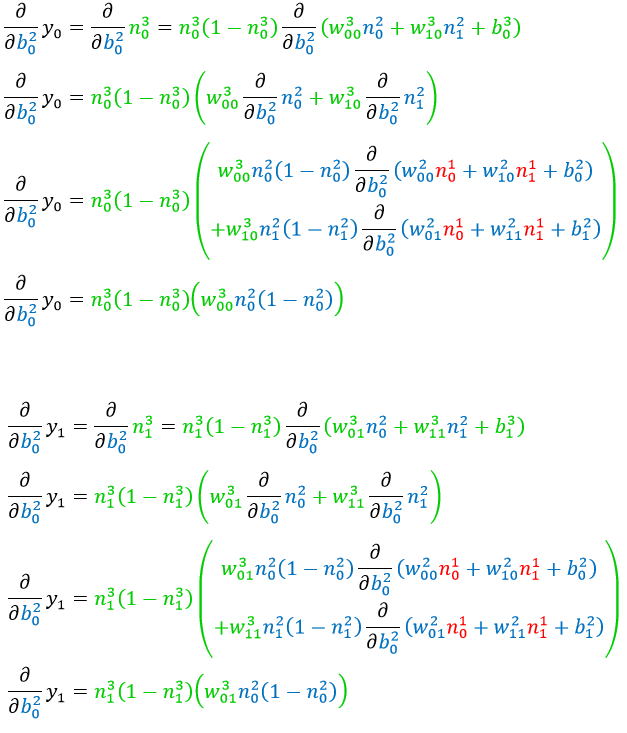And we now plug them back into the original equation.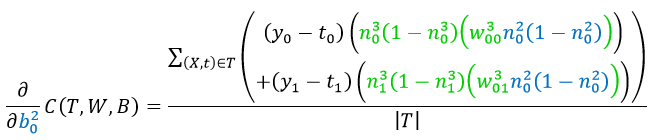Partial derivative with respect to weight in layer 1

Let's find the partial derivative of one of the weights in layer 1 now. We'll skip some steps that were shown in the previous derivations.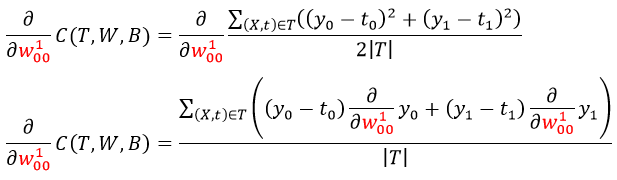And now we find the derivatives of the "y"s separately.And we now plug them back into the original equation.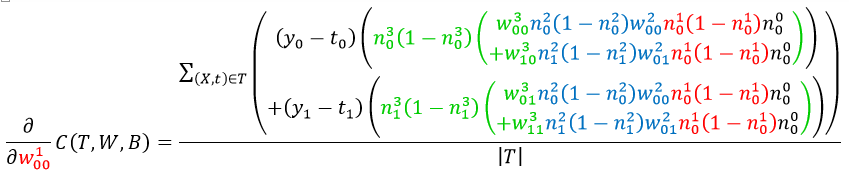Notice that we did not replace the black "n" at layer 0 with "x" in order to preserve a pattern that is revealed later.

Partial derivative with respect to bias in layer 1

Let's find the partial derivative of one of the biases in layer 1 now. We'll skip some steps that were shown in the previous derivations.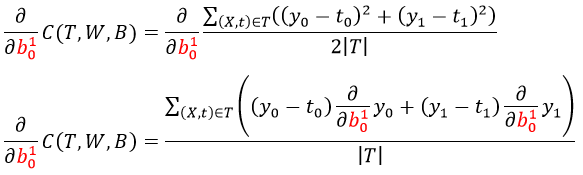And now we find the derivatives of the "y"s separately.And we now plug them back into the original equation.Patterns
There is a pattern in the derivatives. In order to see the pattern, let's look at a part of the equation inside the summations of all the derivatives and compare them.

Here is the repeating pattern in the derivation for the weights:Here is the repeating pattern in the derivation for the biases: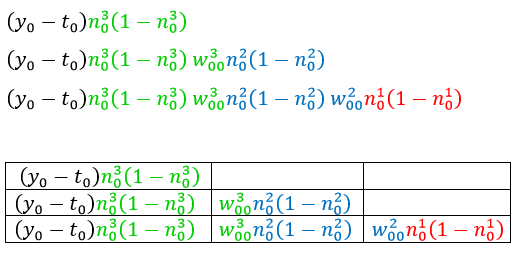See how there is a repeating pattern that gets longer as the layer of the weight we are deriving with respect to gets deeper into the network? This is an important pattern on which the backpropagation algorithm is based, which computes the gradient of a layer's weights using the gradients of the previous layer.

Notice also that as more layers are added, the chain of multiplications gets longer. Since each number is a fraction between 0 and 1, the multiplications produce smaller and smaller numbers as the chain gets longer. This will make the gradients become smaller which makes training the layers at the back very slow. In fact this is a problem in multi-layered neural networks which is known as the vanishing gradient problem. It can be solved by using different activation functions such as the rectified linear unit.

In code
Now that we know how to find the partial derivative of every weight and bias, all we have to do is plug them in the gradient descent algorithm and minimize the cost function. When the cost function is minimized, the network will give us the desired outputs.

Here is a complete code example in Python 3 of a gradient descent algorithm applied to train the above neural network to act as a half adder. A half adder adds together two binary digits and returns the sum and carry. So 0 + 1 in binary gives 1 carry 0 whilst 1 + 1 in binary gives 0 carry 1. One of the functions is called "ns" which gives the outputs of each neuron in the network given an input, grouped by layer. This will be called several times by the gradient functions, so to avoid recomputing the same values, it's output is automatically cached by Python using the lru_cache decorator. The initial values are set to random numbers between 1 and -1 since starting them off as all zeros as was done in the previous blog post will prevent the gradient descent algorithm from working in a neural network.

import math
import random
from functools import lru_cache

trainingset = { (0,0):(0,0), (0,1):(1,0), (1,0):(1,0), (1,1):(0,1) }

old_values = initial_values
while True:
if cost(*new_values) < threshold:
return new_values
old_values = new_values

def a(z):
return 1/(1 + math.exp(-z))

@lru_cache(maxsize=4)
def ns(x0, x1, w100, w101, w110, w111, b10, b11, w200, w201, w210, w211, b20, b21, w300, w301, w310, w311, b30, b31):
(n00, n01) = ( x0, x1 )
(n10, n11) = ( a(n00*w100 + n01*w110 + b10), a(n00*w101 + n01*w111 + b11) )
(n20, n21) = ( a(n10*w200 + n11*w210 + b20), a(n10*w201 + n11*w211 + b21) )
(n30, n31) = ( a(n20*w300 + n21*w310 + b30), a(n20*w301 + n21*w311 + b31) )
return ( (n00, n01), (n10, n11), (n20, n21), (n30, n31) )

def out(x0, x1, w100, w101, w110, w111, b10, b11, w200, w201, w210, w211, b20, b21, w300, w301, w310, w311, b30, b31):
return ns(x0, x1, w100, w101, w110, w111, b10, b11, w200, w201, w210, w211, b20, b21, w300, w301, w310, w311, b30, b31)[-1]

def cost(w100, w101, w110, w111, b10, b11, w200, w201, w210, w211, b20, b21, w300, w301, w310, w311, b30, b31):
tmp = 0
for ((x0,x1), (t0,t1)) in trainingset.items():
(y0, y1) = out(x0, x1, w100, w101, w110, w111, b10, b11, w200, w201, w210, w211, b20, b21, w300, w301, w310, w311, b30, b31)
tmp += (y0 - t0)**2 + (y1 - t1)**2
return tmp/(2*len(trainingset))

def dCdw300(w100, w101, w110, w111, b10, b11, w200, w201, w210, w211, b20, b21, w300, w301, w310, w311, b30, b31):
tmp = 0
for ((x0,x1), (t0,t1)) in trainingset.items():
( (n00, n01), (n10, n11), (n20, n21), (n30, n31) ) = ns(x0, x1, w100, w101, w110, w111, b10, b11, w200, w201, w210, w211, b20, b21, w300, w301, w310, w311, b30, b31)
(y0, y1) = (n30, n31)
tmp += (y0 - t0) * n30*(1-n30) * n20
return tmp/len(trainingset)

def dCdw310(w100, w101, w110, w111, b10, b11, w200, w201, w210, w211, b20, b21, w300, w301, w310, w311, b30, b31):
tmp = 0
for ((x0,x1), (t0,t1)) in trainingset.items():
( (n00, n01), (n10, n11), (n20, n21), (n30, n31) ) = ns(x0, x1, w100, w101, w110, w111, b10, b11, w200, w201, w210, w211, b20, b21, w300, w301, w310, w311, b30, b31)
(y0, y1) = (n30, n31)
tmp += (y0 - t0) * n30*(1-n30) * n21
return tmp/len(trainingset)

def dCdw301(w100, w101, w110, w111, b10, b11, w200, w201, w210, w211, b20, b21, w300, w301, w310, w311, b30, b31):
tmp = 0
for ((x0,x1), (t0,t1)) in trainingset.items():
( (n00, n01), (n10, n11), (n20, n21), (n30, n31) ) = ns(x0, x1, w100, w101, w110, w111, b10, b11, w200, w201, w210, w211, b20, b21, w300, w301, w310, w311, b30, b31)
(y0, y1) = (n30, n31)
tmp += (y1 - t1) * n31*(1-n31) * n20
return tmp/len(trainingset)

def dCdw311(w100, w101, w110, w111, b10, b11, w200, w201, w210, w211, b20, b21, w300, w301, w310, w311, b30, b31):
tmp = 0
for ((x0,x1), (t0,t1)) in trainingset.items():
( (n00, n01), (n10, n11), (n20, n21), (n30, n31) ) = ns(x0, x1, w100, w101, w110, w111, b10, b11, w200, w201, w210, w211, b20, b21, w300, w301, w310, w311, b30, b31)
(y0, y1) = (n30, n31)
tmp += (y1 - t1) * n31*(1-n31) * n21
return tmp/len(trainingset)

def dCdb30(w100, w101, w110, w111, b10, b11, w200, w201, w210, w211, b20, b21, w300, w301, w310, w311, b30, b31):
tmp = 0
for ((x0,x1), (t0,t1)) in trainingset.items():
( (n00, n01), (n10, n11), (n20, n21), (n30, n31) ) = ns(x0, x1, w100, w101, w110, w111, b10, b11, w200, w201, w210, w211, b20, b21, w300, w301, w310, w311, b30, b31)
(y0, y1) = (n30, n31)
tmp += (y0 - t0) * n30*(1-n30)
return tmp/len(trainingset)

def dCdb31(w100, w101, w110, w111, b10, b11, w200, w201, w210, w211, b20, b21, w300, w301, w310, w311, b30, b31):
tmp = 0
for ((x0,x1), (t0,t1)) in trainingset.items():
( (n00, n01), (n10, n11), (n20, n21), (n30, n31) ) = ns(x0, x1, w100, w101, w110, w111, b10, b11, w200, w201, w210, w211, b20, b21, w300, w301, w310, w311, b30, b31)
(y0, y1) = (n30, n31)
tmp += (y1 - t1) * n31*(1-n31)
return tmp/len(trainingset)

def dCdw200(w100, w101, w110, w111, b10, b11, w200, w201, w210, w211, b20, b21, w300, w301, w310, w311, b30, b31):
tmp = 0
for ((x0,x1), (t0,t1)) in trainingset.items():
( (n00, n01), (n10, n11), (n20, n21), (n30, n31) ) = ns(x0, x1, w100, w101, w110, w111, b10, b11, w200, w201, w210, w211, b20, b21, w300, w301, w310, w311, b30, b31)
(y0, y1) = (n30, n31)
tmp += (y0 - t0) * ( n30*(1-n30) * ( w300*n20*(1-n20)*n10 ))
tmp += (y1 - t1) * ( n31*(1-n31) * ( w301*n20*(1-n20)*n10 ))
return tmp/len(trainingset)

def dCdw210(w100, w101, w110, w111, b10, b11, w200, w201, w210, w211, b20, b21, w300, w301, w310, w311, b30, b31):
tmp = 0
for ((x0,x1), (t0,t1)) in trainingset.items():
( (n00, n01), (n10, n11), (n20, n21), (n30, n31) ) = ns(x0, x1, w100, w101, w110, w111, b10, b11, w200, w201, w210, w211, b20, b21, w300, w301, w310, w311, b30, b31)
(y0, y1) = (n30, n31)
tmp += (y0 - t0) * ( n30*(1-n30) * ( w300*n20*(1-n20)*n11 ))
tmp += (y1 - t1) * ( n31*(1-n31) * ( w301*n20*(1-n20)*n11 ))
return tmp/len(trainingset)

def dCdw201(w100, w101, w110, w111, b10, b11, w200, w201, w210, w211, b20, b21, w300, w301, w310, w311, b30, b31):
tmp = 0
for ((x0,x1), (t0,t1)) in trainingset.items():
( (n00, n01), (n10, n11), (n20, n21), (n30, n31) ) = ns(x0, x1, w100, w101, w110, w111, b10, b11, w200, w201, w210, w211, b20, b21, w300, w301, w310, w311, b30, b31)
(y0, y1) = (n30, n31)
tmp += (y0 - t0) * ( n30*(1-n30) * ( w310*n21*(1-n21)*n10 ))
tmp += (y1 - t1) * ( n31*(1-n31) * ( w311*n21*(1-n21)*n10 ))
return tmp/len(trainingset)

def dCdw211(w100, w101, w110, w111, b10, b11, w200, w201, w210, w211, b20, b21, w300, w301, w310, w311, b30, b31):
tmp = 0
for ((x0,x1), (t0,t1)) in trainingset.items():
( (n00, n01), (n10, n11), (n20, n21), (n30, n31) ) = ns(x0, x1, w100, w101, w110, w111, b10, b11, w200, w201, w210, w211, b20, b21, w300, w301, w310, w311, b30, b31)
(y0, y1) = (n30, n31)
tmp += (y0 - t0) * ( n30*(1-n30) * ( w310*n21*(1-n21)*n11 ))
tmp += (y1 - t1) * ( n31*(1-n31) * ( w311*n21*(1-n21)*n11 ))
return tmp/len(trainingset)

def dCdb20(w100, w101, w110, w111, b10, b11, w200, w201, w210, w211, b20, b21, w300, w301, w310, w311, b30, b31):
tmp = 0
for ((x0,x1), (t0,t1)) in trainingset.items():
( (n00, n01), (n10, n11), (n20, n21), (n30, n31) ) = ns(x0, x1, w100, w101, w110, w111, b10, b11, w200, w201, w210, w211, b20, b21, w300, w301, w310, w311, b30, b31)
(y0, y1) = (n30, n31)
tmp += (y0 - t0) * ( n30*(1-n30) * ( w300*n20*(1-n20) ))
tmp += (y1 - t1) * ( n31*(1-n31) * ( w301*n20*(1-n20) ))
return tmp/len(trainingset)

def dCdb21(w100, w101, w110, w111, b10, b11, w200, w201, w210, w211, b20, b21, w300, w301, w310, w311, b30, b31):
tmp = 0
for ((x0,x1), (t0,t1)) in trainingset.items():
( (n00, n01), (n10, n11), (n20, n21), (n30, n31) ) = ns(x0, x1, w100, w101, w110, w111, b10, b11, w200, w201, w210, w211, b20, b21, w300, w301, w310, w311, b30, b31)
(y0, y1) = (n30, n31)
tmp += (y0 - t0) * ( n30*(1-n30) * ( w310*n21*(1-n21) ))
tmp += (y1 - t1) * ( n31*(1-n31) * ( w311*n21*(1-n21) ))
return tmp/len(trainingset)

def dCdw100(w100, w101, w110, w111, b10, b11, w200, w201, w210, w211, b20, b21, w300, w301, w310, w311, b30, b31):
tmp = 0
for ((x0,x1), (t0,t1)) in trainingset.items():
( (n00, n01), (n10, n11), (n20, n21), (n30, n31) ) = ns(x0, x1, w100, w101, w110, w111, b10, b11, w200, w201, w210, w211, b20, b21, w300, w301, w310, w311, b30, b31)
(y0, y1) = (n30, n31)
tmp += (y0 - t0) * ( n30*(1-n30) * ( w300*n20*(1-n20)*w200*n10*(1-n10)*n00 + w310*n21*(1-n21)*w201*n10*(1-n10)*n00 ))
tmp += (y1 - t1) * ( n31*(1-n31) * ( w301*n20*(1-n20)*w200*n10*(1-n10)*n00 + w311*n21*(1-n21)*w201*n10*(1-n10)*n00 ))
return tmp/len(trainingset)

def dCdw110(w100, w101, w110, w111, b10, b11, w200, w201, w210, w211, b20, b21, w300, w301, w310, w311, b30, b31):
tmp = 0
for ((x0,x1), (t0,t1)) in trainingset.items():
( (n00, n01), (n10, n11), (n20, n21), (n30, n31) ) = ns(x0, x1, w100, w101, w110, w111, b10, b11, w200, w201, w210, w211, b20, b21, w300, w301, w310, w311, b30, b31)
(y0, y1) = (n30, n31)
tmp += (y0 - t0) * ( n30*(1-n30) * ( w300*n20*(1-n20)*w200*n10*(1-n10)*n01 + w310*n21*(1-n21)*w201*n10*(1-n10)*n01 ))
tmp += (y1 - t1) * ( n31*(1-n31) * ( w301*n20*(1-n20)*w200*n10*(1-n10)*n01 + w311*n21*(1-n21)*w201*n10*(1-n10)*n01 ))
return tmp/len(trainingset)

def dCdw101(w100, w101, w110, w111, b10, b11, w200, w201, w210, w211, b20, b21, w300, w301, w310, w311, b30, b31):
tmp = 0
for ((x0,x1), (t0,t1)) in trainingset.items():
( (n00, n01), (n10, n11), (n20, n21), (n30, n31) ) = ns(x0, x1, w100, w101, w110, w111, b10, b11, w200, w201, w210, w211, b20, b21, w300, w301, w310, w311, b30, b31)
(y0, y1) = (n30, n31)
tmp += (y0 - t0) * ( n30*(1-n30) * ( w300*n20*(1-n20)*w210*n11*(1-n11)*n00 + w310*n21*(1-n21)*w211*n11*(1-n11)*n00 ))
tmp += (y1 - t1) * ( n31*(1-n31) * ( w301*n20*(1-n20)*w210*n11*(1-n11)*n00 + w311*n21*(1-n21)*w211*n11*(1-n11)*n00 ))
return tmp/len(trainingset)

def dCdw111(w100, w101, w110, w111, b10, b11, w200, w201, w210, w211, b20, b21, w300, w301, w310, w311, b30, b31):
tmp = 0
for ((x0,x1), (t0,t1)) in trainingset.items():
( (n00, n01), (n10, n11), (n20, n21), (n30, n31) ) = ns(x0, x1, w100, w101, w110, w111, b10, b11, w200, w201, w210, w211, b20, b21, w300, w301, w310, w311, b30, b31)
(y0, y1) = (n30, n31)
tmp += (y0 - t0) * ( n30*(1-n30) * ( w300*n20*(1-n20)*w210*n11*(1-n11)*n01 + w310*n21*(1-n21)*w211*n11*(1-n11)*n01 ))
tmp += (y1 - t1) * ( n31*(1-n31) * ( w301*n20*(1-n20)*w210*n11*(1-n11)*n01 + w311*n21*(1-n21)*w211*n11*(1-n11)*n01 ))
return tmp/len(trainingset)

def dCdb10(w100, w101, w110, w111, b10, b11, w200, w201, w210, w211, b20, b21, w300, w301, w310, w311, b30, b31):
tmp = 0
for ((x0,x1), (t0,t1)) in trainingset.items():
( (n00, n01), (n10, n11), (n20, n21), (n30, n31) ) = ns(x0, x1, w100, w101, w110, w111, b10, b11, w200, w201, w210, w211, b20, b21, w300, w301, w310, w311, b30, b31)
(y0, y1) = (n30, n31)
tmp += (y0 - t0) * ( n30*(1-n30) * ( w300*n20*(1-n20)*w200*n10*(1-n10) + w310*n21*(1-n21)*w201*n10*(1-n10) ))
tmp += (y1 - t1) * ( n31*(1-n31) * ( w301*n20*(1-n20)*w200*n10*(1-n10) + w311*n21*(1-n21)*w201*n10*(1-n10) ))
return tmp/len(trainingset)

def dCdb11(w100, w101, w110, w111, b10, b11, w200, w201, w210, w211, b20, b21, w300, w301, w310, w311, b30, b31):
tmp = 0
for ((x0,x1), (t0,t1)) in trainingset.items():
( (n00, n01), (n10, n11), (n20, n21), (n30, n31) ) = ns(x0, x1, w100, w101, w110, w111, b10, b11, w200, w201, w210, w211, b20, b21, w300, w301, w310, w311, b30, b31)
(y0, y1) = (n30, n31)
tmp += (y0 - t0) * ( n30*(1-n30) * ( w300*n20*(1-n20)*w210*n11*(1-n11) + w310*n21*(1-n21)*w211*n11*(1-n11) ))
tmp += (y1 - t1) * ( n31*(1-n31) * ( w301*n20*(1-n20)*w210*n11*(1-n11) + w311*n21*(1-n21)*w211*n11*(1-n11) ))
return tmp/len(trainingset)

cost,
[ dCdw100, dCdw101, dCdw110, dCdw111, dCdb10, dCdb11, dCdw200, dCdw201, dCdw210, dCdw211, dCdb20, dCdb21, dCdw300, dCdw301, dCdw310, dCdw311, dCdb30, dCdb31 ],
[ 2*random.random()-1 for _ in range(18) ],
0.5,
1e-3
)
print('cost:', cost(*new_values))
print('output:')
for ((x0,x1), (t0,t1)) in trainingset.items():
print(' ', (x0,x1), out(x0,x1,*new_values))


Wait a few seconds and...
cost: 0.0009999186077385778
output:
(0, 1) (0.9625083358870274, 0.02072955340806345)
(1, 0) (0.9649753465406843, 0.02060601544190889)
(0, 0) (0.03678639641748687, 0.0051592625464787585)
(1, 1) (0.04304611235264617, 0.9642249998318806)

Conclusion
Of course this isn't a very convenient way to train neural networks since we need to create a different set of partial derivatives for every new network shape (number of neurons and layers). In fact, in a future blog post we'll see how to use the backpropagation algorithm which exploits patterns in this process in order to simplify the process. On the other hand, the backpropagation algorithm makes certain assumptions about the network, such as that every neuron in a layer is connected to every neuron in the previous layer. If we were to make no assumptions about the network then we'd end up using the bare bones method presented here.